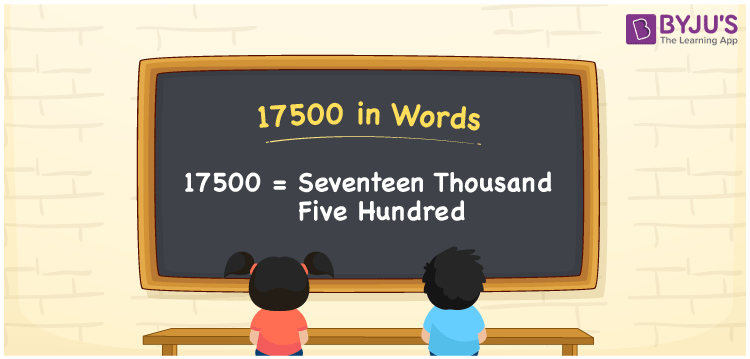# 17500 in words

17500 in words is written as Seventeen Thousand Five Hundred. 17500 represents the count or value. The article on Counting Numbers can give you an idea about count or counting. The number 17500 is used in expressions that relate to money, distance, length and others. Let us consider an example of a crowd at a ground. “There were 17500 seats arranged in the ground for a gathering and all the Seventeen Thousand Five Hundred seats were occupied.”

 17500 in words Seventeen Thousand Five Hundred Seventeen Thousand Five Hundred in Numbers 17500

## 17500 in English Words## How to Write 17500 in Words?

We can convert 17500 to words using a place value chart. The number 17500 has 5 digits, so let’s make a chart that shows the place value up to 5 digits.

 Ten thousand Thousands Hundreds Tens Ones 1 7 5 0 0

Thus, we can write the expanded form as:

1 × Ten thousand + 7 × Thousand + 5 × Hundred + 0 × Ten + 0 × One

= 1 × 10000 + 7 × 1000 + 5 × 100 + 0 × 10 + 0 × 1

= 17500

= Seventeen Thousand Five Hundred

17500 is the natural number that is succeeded by 17499 and preceded by 17501.

17500 in words – Seventeen Thousand Five Hundred.

Is 17500 an odd number? – No.

Is 17500 an even number? – Yes.

Is 17500 a perfect square number? – No.

Is 17500 a perfect cube number? – No.

Is 17500 a prime number? – No.

Is 17500 a composite number? – Yes.

## Solved Example

1. Write the number 17500 in expanded form

Solution: 1 x 10000 + 7 x 1000 + 5 x 100 + 0 x 10 + 0 x 1

Or Just 1 x 10000 + 7 x 1000 + 5 x 100

We can write 17500 = 10000 + 7000 + 500 + 0 + 0

= 1 x 10000 + 7 x 1000 + 5 x 100 + 0 x 10 + 0 x 1

## Frequently Asked Questions on 17500 in words

Q1

### How to write 17500 in words?

17500 in words is written as Seventeen Thousand Five Hundred.
Q2

### State True or False. 17500 is divisible by 4?

True. 17500 is divisible by 4.
Q3

### Is 17500 divisible by 10?

Yes. 17500 is divisible by 10. It is also divisible by 2, 4, 5, 10, and 100.